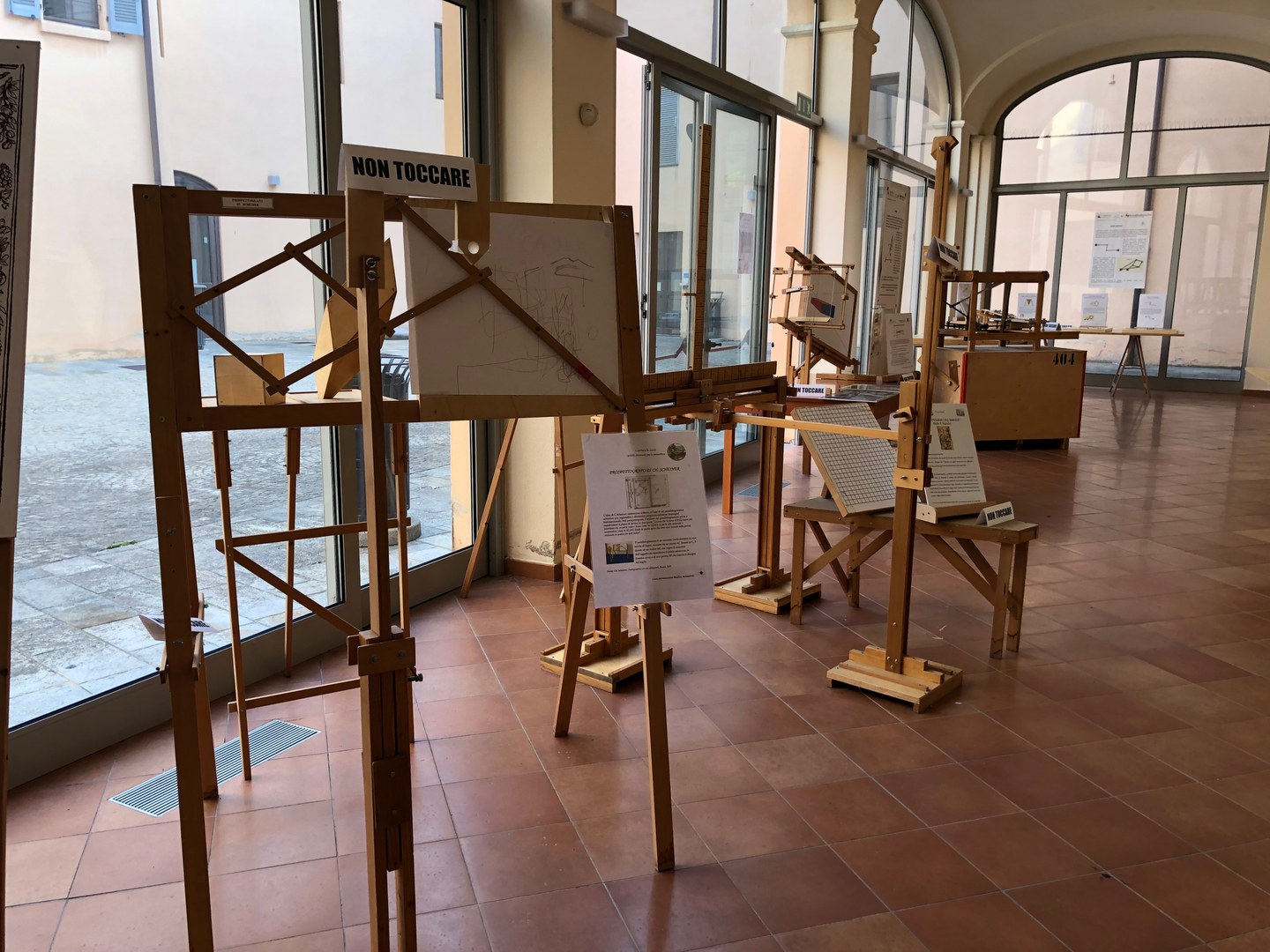Theme ART AND CULTURE

# Laboratory of mathematical machines

The Laboratory of mathematical machines (the MMLab), at the University of Modena e Reggio Emilia,contains a collection of geometrical instruments (called ‘mathematical machines’).

They have been reconstructed with a didactical aim, according to the design described in historical texts from classical Greece (linked to the theory of conic sections) to the 20th century. The MMLab works for both mathematics education research and popularisation of mathematics (above all by the organisation of thematic exhibitions).

It offers mathematics laboratory sessions to primary and secondary school students.

The MMLab staff includes academic researchers, university students, teachers and the members of association ‘Macchine Matematiche’.

What is a mathematical machine?

A mathematical machine (related to the field of geometry) is an artefact designed and built for the following purpose, that does not depend on the practical use (if any): it aims at forcing a point, a line segment or a plane figure (supported by a material support that makes them visible and touchable) to move or to be transformed according to a mathematical law that has been determined by the designer.

The most well-known mathematical machine is the pair of compasses (to draw circles) that is part of the iconography of mathematicians. It is the ancestor of many curve drawing devices and pantographs. Another class of mathematical machines is given by perspectographs that are related to the ancient three-dimensional theory of conics, on the one hand, and to the roots of projective geometry on the other hand.

In the MMLab there are different kinds of instruments:

- curve drawing devices;

- pantographs for geometric transformations;

- models of conic sections;

- models of singular point of algebraic curves;

- models of the 3D genesis of plane geometric transformations;

- perspectographs;

- anamorphoses.

Permanent exhibition in via Camatta 15, Modena.